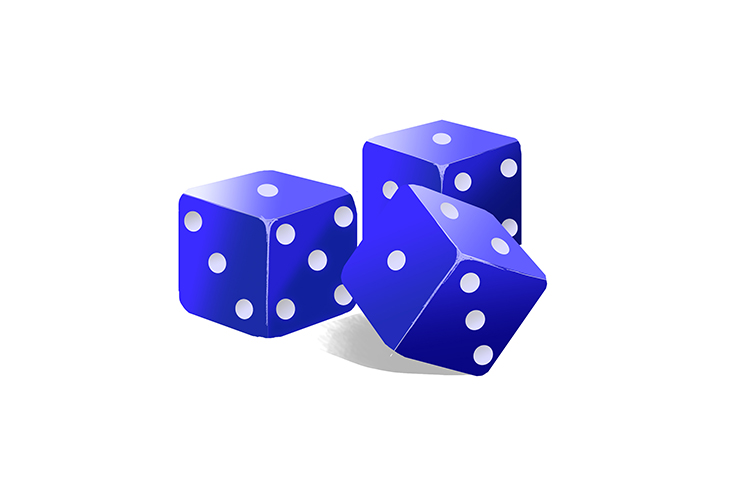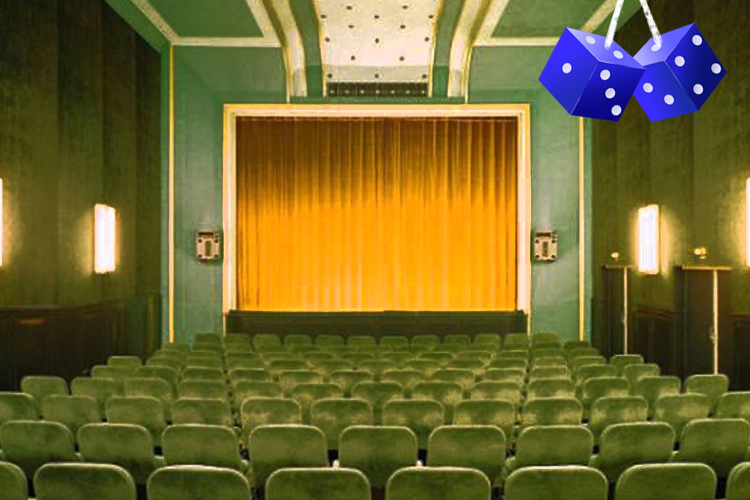# Probability

## Probability is the chance that something will happen

Probability = (The\ n\umber\ of\ ways\ of\ ach\ievi\ng\ suc\c\ess)/(The\ n\umber\ of\ possibl\e\ outcomes

Or put more simply

Probability = (N\umber\ of\ RIGHT\ answers)/(The\ n\umber\ of\ALL\ answersIf probability is represented by dice then:The dice (probability) was on the RIGHT (right) above the Hall (all).

Probability = (RIGHT)/(ALL)